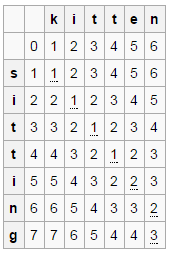# The Levenshtein distance (Edit distance) problem

Edit distance is a way of quantifying how dissimilar two strings are to one another by counting the minimum number of operations required to transform one string into the other.

The Levenshtein distance between two words is the minimum number of single-character edits (i.e. insertions, deletions or substitutions) required to change one word into the other. Each of these operations has unit cost.

For example, the Levenshtein distance between kitten and sitting is 3. A minimal edit script that transforms the former into the latter is:

kitten -> sitten (substitution of s for k)
sitten -> sittin (substitution of i for e)
sittin -> sitting (insertion of g at the end)

The Edit distance problem has an optimal substructure. That means the problem can be broken down into smaller, simple “subproblems”, which can be broken down into yet simpler subproblems, and so on, until, finally, the solution becomes trivial.

Problem: Convert string X[1..m] to Y[1..n] by performing edit operations on string X.

Sub-problem: Convert substring X[1..i] to Y[1..j] by performing edit operations on substring X.

CASE 1: We have reached the end of either substring

If substring X is empty, then we insert all remaining characters of substring Y to X and the cost of this operation is equal to number of characters left in substring Y.

('', 'ABC') --> ('ABC', 'ABC') (cost = 3)

If substring Y is empty, then we delete all remaining characters of X to convert it into substring Y. The cost of this operation is equal to number of characters left in substring X.

('ABC', '') --> ('', '') (cost = 3)

CASE 2: Last characters of substring X and substring Y are same

If last characters of substring X and substring Y matches, nothing needs to be done. We simply recurse for remaining substring X[0..i-1], Y[0..j-1]. As no edit operation is involved, the cost will be 0.

('ACC', 'ABC') --> ('AC', 'AB') (cost = 0)

CASE 3: Last characters of substring X and substring Y are different

If the last characters of substring X and substring Y are different, then we return minimum of below three operations –

• Insert last character of Y to X. The size of Y reduces by 1 and size of X remains the same. This accounts for X[1..i], Y[1..j-1] as we move in on target substring, but not in source substring.

('ABA', 'ABC') --> ('ABAC', 'ABC') ==  ('ABA', 'AB') (using case 2)

•

• Delete last character of X. The size of X reduces by 1 and size of Y remains the same. This accounts for X[1..i-1], Y[1..j] as we move in on source string, but not in target string.

('ABA', 'ABC') --> ('AB', 'ABC')

•

• Substitute (Replace) current character of X by current character of Y. The size of both X and Y reduces by 1. This accounts for X[1..i-1], Y[1..j-1] as we move in both source string and target string.

('ABA', 'ABC') - > ('ABC', 'ABC')  == ('AB', 'AB')  (using case 2)

It is basically same as case 2 where the last two characters matches and we move in both source string and target string except it costs an edit operation.

So we can define the problem recursively as –

| max(i, j)                       when min(i, j) = 0

dist[i][j] = | dist[i - 1][j - 1]              when X[i-1] == Y[j-1]

| 1 + minimum { dist[i - 1][j],   when X[i-1] != Y[j-1]
|               dist[i][j - 1],
|               dist[i - 1][j - 1] }

Below is C++ and Java implementation of the idea:

## C++

Output:

The Levenshtein Distance is 3

## Java

Output:

The Levenshtein Distance is 3

The time complexity of above solution is O(3n) and auxiliary space used by the program is constant.

As seen above, the problem has an optimal substructure. Above solution also exhibits overlapping subproblems. If we draw the recursion tree of the solution, we can see that the same sub-problems are getting computed again and again. We know that problems having optimal substructure and overlapping subproblems can be solved by using dynamic programming, in which subproblem solutions are memoized rather than computed again and again.

The Memoized version follows the top-down approach, since we first break the problem into subproblems and then calculate and store values. We can also solve this problem in bottom-up manner. In the bottom-up approach, we solve smaller sub-problems first, then solve larger sub-problems from them.

The invariant maintained throughout the algorithm is that we can transform the initial segment X[1..i] into Y[1..j] using a minimum of T[i,j] operations. At the end, the bottom-right element of the array contains the answer.

For example, let X be kitten and Y be sitting. The Levenshtein distance between X and Y is 3. The ith row and jth column in the table below shows the Levenshtein distance of substring X[0..i-1] and Y[0..j-1].Below is C++ and Java implementation of the idea:

## C++

Output:

The Levenshtein Distance is 3

## Java

Output:

The Levenshtein Distance is 3

The time complexity of above solution is O(mn) and auxiliary space used by the program is O(mn) where m and n are the number of characters in two strings. It turns out that only two rows of the table are needed for the construction if one does not want to reconstruct the edited input strings (the previous row and the current row being calculated).

Exercise: Modify Iterative version to use only two matrix rows.

References: Levenshtein Distance – Wikipedia(1 votes, average: 5.00 out of 5)Loading...